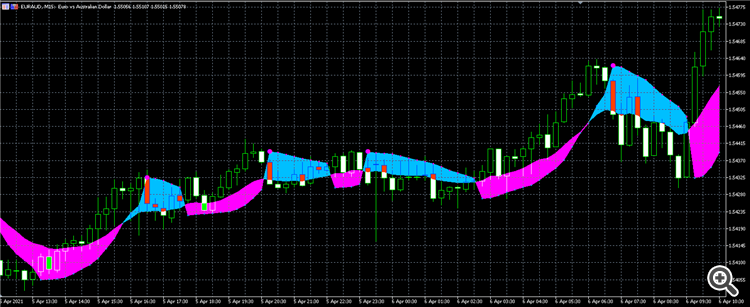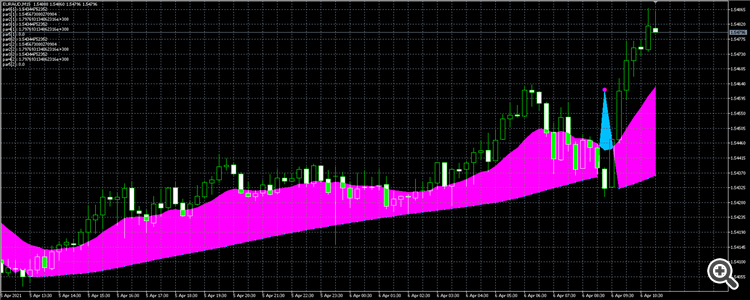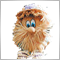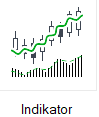# Indicator deviates in the strategy tester177

Hey guy,

i want to use this indicator in my EA. Below you can see a picture of the indicator in any chart:Now a picture from the same chart, but within the backtester:I´ve used 1m OHLC and tick data as well. nothing works. In the left upper corner you can see all the buffers. This values are extremely. Maybe there is something wrong but i dont get it..
The indicators file is attached as well as the code. Thanks for your time.

```
input int III = 1;// ~~~~~~~~~~~~~~~~~~~ParabolicUsMoving~~~~~~~~~~~~~~~~
input int   MAPeriod=13;
input  ENUM_MA_METHOD   MAType=MODE_EMA;
input ENUM_APPLIED_PRICE   MAPrice=PRICE_CLOSE;
input double StepH_=0.02;//Шаг для верхних точек
input double MaximumH=0.5;//Максимум для верхних точек
input double StepL_=0.02;//Шаг для нижних точек
input double MaximumL=0.5;//Максимум для нижних точек

int parabHandle;

double  sl11;
double par0[];
double par1[];
double par2[];
double par3[];
double par4[];
double par5[];

int OnInit()
{

parabHandle= iCustom(_Symbol,PERIOD_M15,"AAA_EA_Indicators\\Trigger\\ParabolicUsMoving",MAPeriod,MAType,MAPrice,StepH_,StepL_,MaximumH,MaximumL);

return(INIT_SUCCEEDED);
}

void OnDeinit(const int reason)
{

}

void OnTick()
{

CopyBuffer(parabHandle,0,1,3,par0);
CopyBuffer(parabHandle,1,1,3,par1);
CopyBuffer(parabHandle,2,1,3,par2);
CopyBuffer(parabHandle,3,1,3,par3);
CopyBuffer(parabHandle,4,1,3,par4);
CopyBuffer(parabHandle,5,1,3,par5);

ArraySetAsSeries(par0,true);
ArraySetAsSeries(par1,true);
ArraySetAsSeries(par2,true);
ArraySetAsSeries(par3,true);
ArraySetAsSeries(par4,true);
ArraySetAsSeries(par5,true);

Comment("par0: ",par0,
"\npar1: ",par1,
"\npar2: ",par2,
"\npar3: ",par3,
"\npar4: ",par4,
"\npar5: ",par5,
"\npar0: ",par0,
"\npar1: ",par1,
"\npar2: ",par2,
"\npar3: ",par3,
"\npar4: ",par4,
"\npar5: ",par5);
```
Files:Moderator
246808

You need to look at the indicator code - you just have to know what is in each buffer. Only then can the information be copied from the buffer.177

yeah, i know what you mean, but even if i just call the indicators handle, the chart looks exactly the same like the example above.

oddly enough, when I am running the indikator by choosing this mode:it works perfectly fineModerator
246808

steyr6155 :
yeah, i know what you mean, but even if i just call the indicators handle, the chart looks exactly the same like the example above.

oddly enough, when I am running the indikator by choosing this mode:

it works perfectly fine

Where is the indicator code?177

I have also added the mql5 file in my first post:

```//+---------------------------------------------------------------------+
//|                                               ParabolicUsMoving.mq5 |
//|                                  Copyright © 2017, Nikolay Kositsin |
//|                                 Khabarovsk,   farria@mail.redcom.ru |
//+---------------------------------------------------------------------+
#property copyright "Copyright © 2017, Nikolay Kositsin"
#property link "farria@mail.redcom.ru"
//---- номер версии индикатора
#property version   "1.00"
//---- отрисовка индикатора в главном окне
#property indicator_chart_window
//---- для расчёта и отрисовки индикатора использовано шесть буферов
#property indicator_buffers 6
//---- использовано всего пять графических построений
#property indicator_plots   4
//+----------------------------------------------+
//|  Параметры отрисовки индикатора 1            |
//+----------------------------------------------+
//--- отрисовка индикатора в виде цветного облака
#property indicator_type1   DRAW_FILLING
//--- в качестве цветов индикатора использованы
#property indicator_color1  clrDeepSkyBlue,clrMagenta
//--- отображение метки индикатора
#property indicator_label1  "Signal HTF"
//+----------------------------------------------+
//|  Параметры отрисовки медвежьего индикатора   |
//+----------------------------------------------+
//---- отрисовка индикатора 2 в виде символа
#property indicator_type2   DRAW_ARROW
//---- в качестве цвета индикатора использован Magenta цвет
#property indicator_color2  clrMagenta
//---- толщина индикатора 2 равна 2
#property indicator_width2  2
//---- отображение бычей метки индикатора
#property indicator_label2  "Lower Parabolic"
//+----------------------------------------------+
//|  Параметры отрисовки бычьго индикатора       |
//+----------------------------------------------+
//---- отрисовка индикатора 3 в виде символа
#property indicator_type3   DRAW_ARROW
//---- в качестве цвета индикатора использован DodgerBlue цвет
#property indicator_color3  clrDodgerBlue
//---- толщина индикатора 2 равна 2
#property indicator_width3  2
//---- отображение медвежьей метки индикатора
#property indicator_label3 "Upper Parabolic"
//+----------------------------------------------+
//|  Параметры отрисовки медвежьего индикатора   |
//+----------------------------------------------+
//---- отрисовка индикатора 4 в виде символа
#property indicator_type4   DRAW_ARROW
//---- в качестве цвета индикатора использован Magenta цвет
#property indicator_color4  clrMagenta
//---- толщина индикатора 4 равна 4
#property indicator_width4  4
//---- отображение бычей метки индикатора
#property indicator_label4  "Parabolic Sell"
//+----------------------------------------------+
//|  Параметры отрисовки бычьго индикатора       |
//+----------------------------------------------+
//---- отрисовка индикатора 5 в виде символа
#property indicator_type5   DRAW_ARROW
//---- в качестве цвета индикатора использован DodgerBlue цвет
#property indicator_color5  clrDodgerBlue
//---- толщина индикатора 5 равна 4
#property indicator_width5  4
//---- отображение медвежьей метки индикатора
#property indicator_label5 "Parabolic Buy"
//+----------------------------------------------+
//|  объявление констант                         |
//+----------------------------------------------+
#define RESET  0 // Константа для возврата терминалу команды на пересчёт индикатора
//+----------------------------------------------+
//| Входные параметры индикатора                 |
//+----------------------------------------------+
//---- Параметры индикатора Parabolic
input int   MAPeriod=13;
input  ENUM_MA_METHOD   MAType=MODE_EMA;
input ENUM_APPLIED_PRICE   MAPrice=PRICE_CLOSE;
input double StepH_=0.02;//Шаг для верхних точек
input double MaximumH=0.5;//Максимум для верхних точек
input double StepL_=0.02;//Шаг для нижних точек
input double MaximumL=0.5;//Максимум для нижних точек
//+----------------------------------------------+

//---- объявление динамических массивов, которые будут в дальнейшем использованы в качестве индикаторных буферов
double BuyBuffer[],SellBuffer[];
double UpSarBuffer[],DnSarBuffer[];
double SarBuffer[],LineBuffer[];
//----
bool dirlong_,first_;
double ep_,start_,last_high_,last_low_,prev_sar_;
double StepH,StepL;
//---- Объявление целых переменных для хендлов индикаторов
int MA_Handle;
//---- Объявление целых переменных начала отсчёта данных
int min_rates_total;
//+------------------------------------------------------------------+
//| Parabolic indicator initialization function                      |
//+------------------------------------------------------------------+
int OnInit()
{
//---- Инициализация переменных начала отсчёта данных
min_rates_total=MAPeriod+2;
StepH=MathMin(StepH_,MaximumH);
StepL=MathMin(StepL_,MaximumL);
//---- получение хендла индикатора iMA
MA_Handle=iMA(NULL,0,MAPeriod,0,MAType,MAPrice);
if(MA_Handle==INVALID_HANDLE)
{
Print(" Не удалось получить хендл индикатора iMA");
return(INIT_FAILED);
}
//---- превращение динамического массива в индикаторный буфер
SetIndexBuffer(0,SarBuffer,INDICATOR_DATA);
//---- осуществление сдвига начала отсчёта отрисовки индикатора 1
PlotIndexSetInteger(0,PLOT_DRAW_BEGIN,min_rates_total);
//---- символ для индикатора
PlotIndexSetInteger(0,PLOT_ARROW,158);
//---- установка значений индикатора, которые не будут видимы на графике
PlotIndexSetDouble(0,PLOT_EMPTY_VALUE,EMPTY_VALUE);

//---- превращение динамического массива в индикаторный буфер
SetIndexBuffer(1,LineBuffer,INDICATOR_DATA);

//---- превращение динамического массива в индикаторный буфер
SetIndexBuffer(2,UpSarBuffer,INDICATOR_DATA);
//---- осуществление сдвига начала отсчёта отрисовки индикатора 1
PlotIndexSetInteger(1,PLOT_DRAW_BEGIN,min_rates_total);
//---- символ для индикатора
PlotIndexSetInteger(1,PLOT_ARROW,158);
//---- установка значений индикатора, которые не будут видимы на графике
PlotIndexSetDouble(1,PLOT_EMPTY_VALUE,EMPTY_VALUE);

//---- превращение динамического массива в индикаторный буфер
SetIndexBuffer(3,DnSarBuffer,INDICATOR_DATA);
//---- осуществление сдвига начала отсчёта отрисовки индикатора 2
PlotIndexSetInteger(2,PLOT_DRAW_BEGIN,min_rates_total);
//---- символ для индикатора
PlotIndexSetInteger(2,PLOT_ARROW,158);
//---- установка значений индикатора, которые не будут видимы на графике
PlotIndexSetDouble(2,PLOT_EMPTY_VALUE,EMPTY_VALUE);

//---- превращение динамического массива в индикаторный буфер
SetIndexBuffer(4,SellBuffer,INDICATOR_DATA);
//---- осуществление сдвига начала отсчёта отрисовки индикатора 3
PlotIndexSetInteger(3,PLOT_DRAW_BEGIN,min_rates_total);
//---- символ для индикатора
PlotIndexSetInteger(3,PLOT_ARROW,159);
//---- установка значений индикатора, которые не будут видимы на графике
PlotIndexSetDouble(3,PLOT_EMPTY_VALUE,EMPTY_VALUE);

//---- превращение динамического массива в индикаторный буфер
SetIndexBuffer(5,BuyBuffer,INDICATOR_DATA);
//---- осуществление сдвига начала отсчёта отрисовки индикатора 4
PlotIndexSetInteger(4,PLOT_DRAW_BEGIN,min_rates_total);
//---- символ для индикатора
PlotIndexSetInteger(4,PLOT_ARROW,159);
//---- установка значений индикатора, которые не будут видимы на графике
PlotIndexSetDouble(4,PLOT_EMPTY_VALUE,EMPTY_VALUE);

//---- создание имени для отображения в отдельном подокне и во всплывающей подсказке
IndicatorSetString(INDICATOR_SHORTNAME,"ParabolicUsMoving");

//---- определение точности отображения значений индикатора
IndicatorSetInteger(INDICATOR_DIGITS,_Digits+1);
//---- завершение инициализации
return(INIT_SUCCEEDED);
}
//+------------------------------------------------------------------+
//| Parabolic iteration function                                     |
//+------------------------------------------------------------------+
int OnCalculate(
const int rates_total,    // количество истории в барах на текущем тике
const int prev_calculated,// количество истории в барах на предыдущем тике
const datetime &time[],
const double &open[],
const double &high[],
const double &low[],
const double &close[],
const long &tick_volume[],
const long &volume[],
const int &spread[]
)
{
//---- проверка количества баров на достаточность для расчёта
if(rates_total<min_rates_total || BarsCalculated(MA_Handle)<rates_total)  return(RESET);

//---- Объявление переменных с плавающей точкой
double price_low,price_high,sar;
double ep,start,last_high,last_low,prev_sar;
//---- Объявление целых переменных и получение уже посчитанных баров
int gfirst,bar,to_copy;
bool dirlong,first;

//---- расчёт стартового номера first для цикла пересчёта баров
if(prev_calculated>rates_total || prev_calculated<=0) // проверка на первый старт расчёта индикатора
{
gfirst=min_rates_total; // стартовый номер для расчёта всех баров
to_copy=rates_total-gfirst+1;
first_=false;
dirlong_=false;
last_high_=0.0;
last_low_=999999999.0;
ep_=PriceSeries(MAPrice,min_rates_total-1,open,low,high,close);
prev_sar_=ep_;
start_=NULL;
}
else
{
gfirst=prev_calculated-1; // стартовый номер для расчёта новых баров
to_copy=rates_total-prev_calculated+1;
}

//---- копируем вновь появившиеся данные в массив
if(CopyBuffer(MA_Handle,0,0,to_copy,LineBuffer)<=0) return(RESET);

//---- восстановим значения переменных
ep=ep_;
start=start_;
last_high=last_high_;
last_low=last_low_;
dirlong=dirlong_;
first=first_;
prev_sar=prev_sar_;

//---- Основной цикл расчёта индикатора Parabolic
for(bar=gfirst; bar<rates_total; bar++)
{
price_low=PriceSeries(MAPrice,bar,open,low,high,close);
price_high=PriceSeries(MAPrice,bar,open,low,high,close);
sar=prev_sar+start*(ep-prev_sar);
//----
if(dirlong)
{
if(ep<price_high && start+StepL<=MaximumL) start+=StepL;
if(sar>=price_low)
{
start=StepL;
dirlong=false;
ep=price_low;
last_low=price_low;

if(price_high<last_high) sar=last_high;
else sar=price_high;
}
else
{
if(ep<price_low && start+StepL<=MaximumL) start+=StepL;

if(ep<price_high)
{
last_high=price_high;
ep=price_high;
}
}
}
//----
else
{
if(ep>price_low && start+StepH<=MaximumH) start+=StepH;
if(sar<=price_high)
{
start=StepH;
dirlong=true;
ep=price_high;
last_high=price_high;
if(price_low>last_low) sar=last_low;
else sar=price_low;
}
else
{
if(ep>price_high && start+StepH<=MaximumH) start+=StepH;

if(ep>price_low)
{
last_low=price_low;
ep=price_low;
}
}
}

SarBuffer[bar]=sar;
//---- обнулим содержимое индикаторных буферов до расчёта
DnSarBuffer[bar]=EMPTY_VALUE;
UpSarBuffer[bar]=EMPTY_VALUE;

if(PriceSeries(MAPrice,bar,open,low,high,close)<sar) UpSarBuffer[bar]=sar;
else DnSarBuffer[bar]=sar;

//---- сохраним значения переменных
if(bar==rates_total-2)
{
ep_=ep;
start_=start;
last_high_=last_high;
last_low_=last_low;
dirlong_=dirlong;
first_=first;
prev_sar_=sar;
}

if(bar<rates_total-1) prev_sar=sar;
}

//---- пересчёт стартового номера для расчёта всех баров
if(prev_calculated>rates_total || prev_calculated<=0)// проверка на первый старт расчёта индикатора
gfirst++;

//---- второй цикл расчёта Parabolic
for(bar=gfirst; bar<rates_total; bar++)
{
//---- обнулим содержимое индикаторных буферов до расчёта
BuyBuffer[bar]=EMPTY_VALUE;
SellBuffer[bar]=EMPTY_VALUE;

if(UpSarBuffer[bar-1]==EMPTY_VALUE&&UpSarBuffer[bar]!=EMPTY_VALUE) SellBuffer[bar]=UpSarBuffer[bar];
if(DnSarBuffer[bar-1]==EMPTY_VALUE&&DnSarBuffer[bar]!=EMPTY_VALUE) BuyBuffer[bar]=DnSarBuffer[bar];
}
//----
return(rates_total);
}
//+------------------------------------------------------------------+
//| Получение значения ценовой таймсерии                             |
//+------------------------------------------------------------------+
double PriceSeries
(
uint applied_price,// Ценовая константа
uint   bar,// Индекс сдвига относительно текущего бара на указанное количество периодов назад или вперёд).
const double &Open[],
const double &Low[],
const double &High[],
const double &Close[]
)
//PriceSeries(applied_price, bar, open, low, high, close)
//+ - - - - - - - - - - - - - - - - - - - - - - - - - - - - - - - - -+
{
//----
switch(applied_price)
{
//---- Ценовые константы из перечисления ENUM_APPLIED_PRICE
case  PRICE_CLOSE: return(Close[bar]);
case  PRICE_OPEN: return(Open [bar]);
case  PRICE_HIGH: return(High [bar]);
case  PRICE_LOW: return(Low[bar]);
case  PRICE_MEDIAN: return((High[bar]+Low[bar])/2.0);
case  PRICE_TYPICAL: return((Close[bar]+High[bar]+Low[bar])/3.0);
case  PRICE_WEIGHTED: return((2*Close[bar]+High[bar]+Low[bar])/4.0);

//----
case  8: return((Open[bar] + Close[bar])/2.0);
case  9: return((Open[bar] + Close[bar] + High[bar] + Low[bar])/4.0);
//----
case 10:
{
if(Close[bar]>Open[bar])return(High[bar]);
else
{
if(Close[bar]<Open[bar])
return(Low[bar]);
else return(Close[bar]);
}
}
//----
case 11:
{
if(Close[bar]>Open[bar])return((High[bar]+Close[bar])/2.0);
else
{
if(Close[bar]<Open[bar])
return((Low[bar]+Close[bar])/2.0);
else return(Close[bar]);
}
break;
}
//----
case 12:
{
double res=High[bar]+Low[bar]+Close[bar];
if(Close[bar]<Open[bar]) res=(res+Low[bar])/2;
if(Close[bar]>Open[bar]) res=(res+High[bar])/2;
if(Close[bar]==Open[bar]) res=(res+Close[bar])/2;
return(((res-Low[bar])+(res-High[bar]))/2);
}
//----
default: return(Close[bar]);
}
//----
//return(0);
}
//+------------------------------------------------------------------+
```